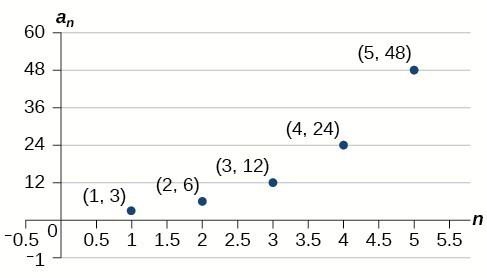## Solutions to Try Its

1. The sequence is not geometric because $\frac{10}{5}\ne \frac{15}{10}$ .

2. The sequence is geometric. The common ratio is $\frac{1}{5}$ .

3. $\left\{18,6,2,\frac{2}{3},\frac{2}{9}\right\}$

4. $\begin{array}{l}{a}_{1}=2\\ {a}_{n}=\frac{2}{3}{a}_{n - 1}\text{ for }n\ge 2\end{array}$

5. ${a}_{6}=16,384$

6. ${a}_{n}=-{\left(-3\right)}^{n - 1}$

7. a. ${P}_{n} = 293\cdot 1.026{a}^{n}$
b. The number of hits will be about 333.

## Solutions to Odd-Numbered Exercises

1. A sequence in which the ratio between any two consecutive terms is constant.

3. Divide each term in a sequence by the preceding term. If the resulting quotients are equal, then the sequence is geometric.

5. Both geometric sequences and exponential functions have a constant ratio. However, their domains are not the same. Exponential functions are defined for all real numbers, and geometric sequences are defined only for positive integers. Another difference is that the base of a geometric sequence (the common ratio) can be negative, but the base of an exponential function must be positive.

7. The common ratio is $-2$

9. The sequence is geometric. The common ratio is 2.

11. The sequence is geometric. The common ratio is $-\frac{1}{2}$.

13. The sequence is geometric. The common ratio is $5$.

15. $5,1,\frac{1}{5},\frac{1}{25},\frac{1}{125}$

17. $800,400,200,100,50$

19. ${a}_{4}=-\frac{16}{27}$

21. ${a}_{7}=-\frac{2}{729}$

23. $7,1.4,0.28,0.056,0.0112$

25. $\begin{array}{cc}a{}_{1}=-32,& {a}_{n}=\frac{1}{2}{a}_{n - 1}\end{array}$

27. $\begin{array}{cc}{a}_{1}=10,& {a}_{n}=-0.3{a}_{n - 1}\end{array}$

29. $\begin{array}{cc}{a}_{1}=\frac{3}{5},& {a}_{n}=\frac{1}{6}{a}_{n - 1}\end{array}$

31. ${a}_{1}=\frac{1}{512},{a}_{n}=-4{a}_{n - 1}$

33. $12,-6,3,-\frac{3}{2},\frac{3}{4}$

35. ${a}_{n}={3}^{n - 1}$

37. ${a}_{n}=0.8\cdot {\left(-5\right)}^{n - 1}$

39. ${a}_{n}=-{\left(\frac{4}{5}\right)}^{n - 1}$

41. ${a}_{n}=3\cdot {\left(-\frac{1}{3}\right)}^{n - 1}$

43. ${a}_{12}=\frac{1}{177,147}$

45. There are $12$ terms in the sequence.

47. The graph does not represent a geometric sequence.

49.51. Answers will vary. Examples: ${\begin{array}{cc}{a}_{1}=800,& {a}_{n}=0.5a\end{array}}_{n - 1}$ and ${\begin{array}{cc}{a}_{1}=12.5,& {a}_{n}=4a\end{array}}_{n - 1}$

53. ${a}_{5}=256b$

55. The sequence exceeds $100$ at the 14th term, ${a}_{14}\approx 107$.

57. ${a}_{4}=-\frac{32}{3}$ is the first non-integer value

59. Answers will vary. Example: Explicit formula with a decimal common ratio: ${a}_{n}=400\cdot {0.5}^{n - 1}$; First 4 terms: $\begin{array}{cc}400,200,100,50;& {a}_{8}=3.125\end{array}$